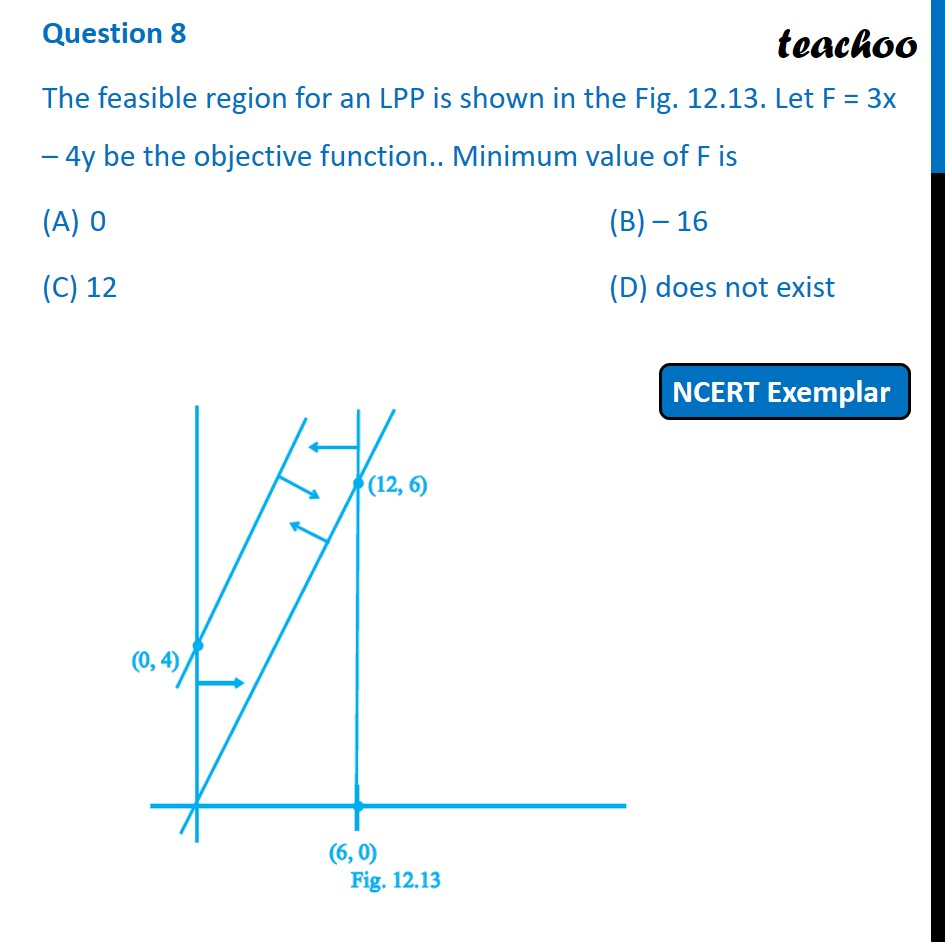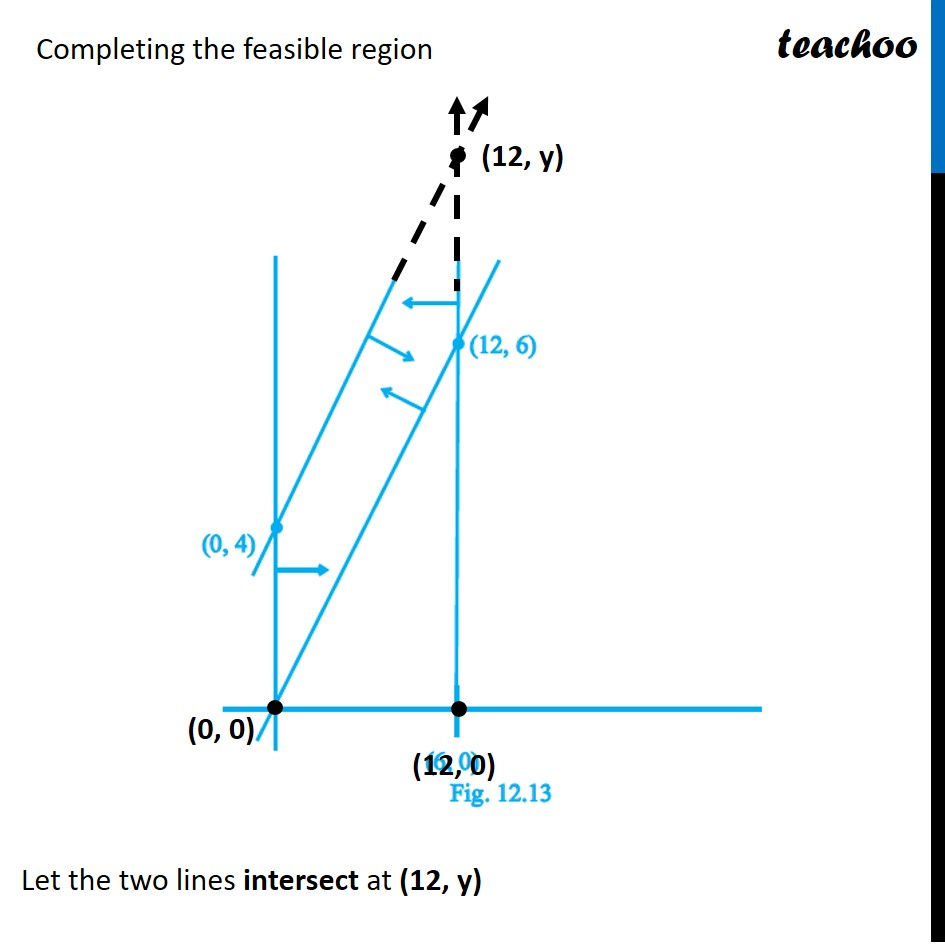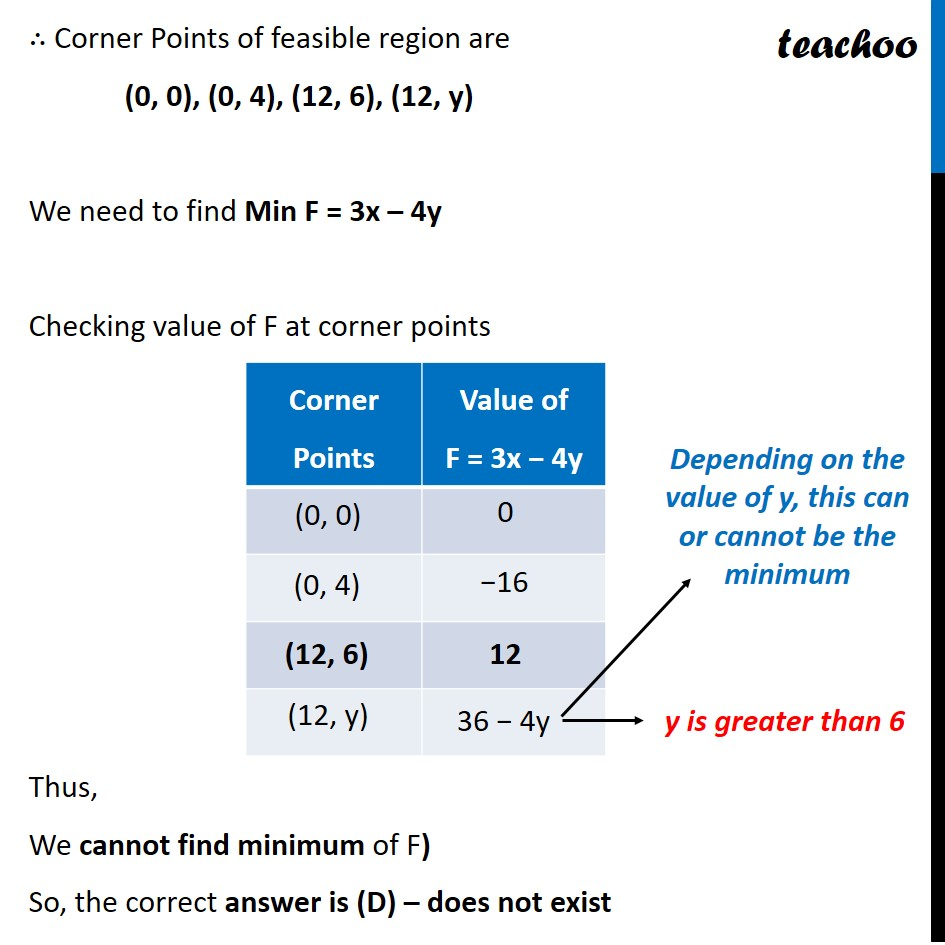NCERT Exemplar - MCQs

Chapter 12 Class 12 Linear Programming
Serial order wise

## (C) 12                          (D) does not exist

This question is similar to Question 38 Choice 2 - Sample Paper 2021 Class 12 - Linear ProgrammingLearn in your speed, with individual attention - Teachoo Maths 1-on-1 Class

### Transcript

Question 8 The feasible region for an LPP is shown in the Fig. 12.13. Let F = 3x – 4y be the objective function.. Minimum value of F is 0 (B) – 16 (C) 12 (D) does not exist Completing the feasible region Let the two lines intersect at (12, y) ∴ Corner Points of feasible region are (0, 0), (0, 4), (12, 6), (12, y) We need to find Min F = 3x – 4y Checking value of F at corner points Thus, We cannot find minimum of F) So, the correct answer is (D) – does not exist